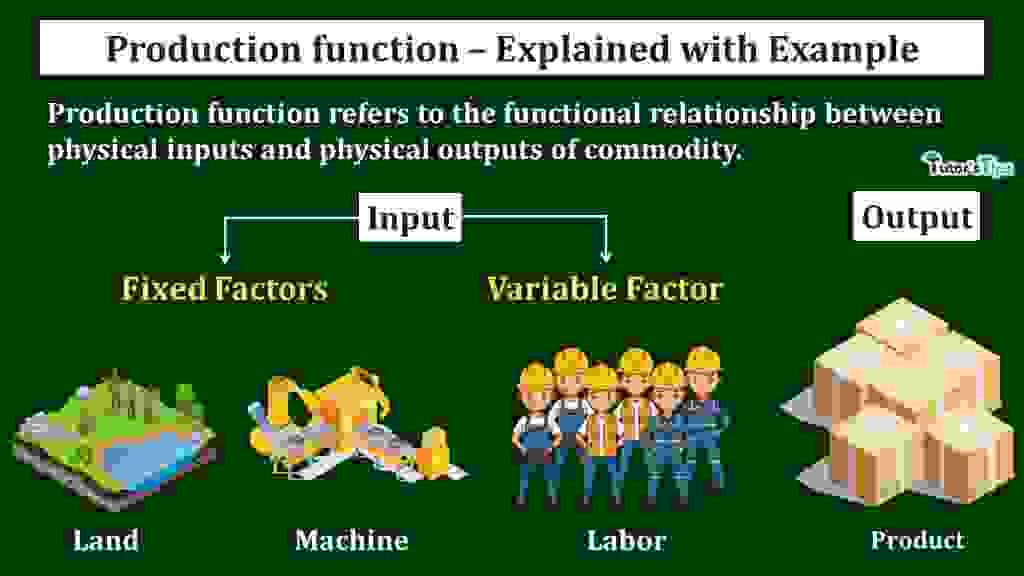# Production Function – Meaning and TypesProduction function refers to the functional relationship between physical inputs and physical outputs of a commodity.

## Concept of Production Function :

As we know, for producing output, we need inputs. Generally, the land, labour and capital are well known and well-organized inputs used for the production of goods and services.

Hence, The producer is always keen to know the number of inputs needed to produce a given quantity of a commodity. For instance, the producer may find that 20 units of capital and 10 units of labour are required to produce 200 units of a commodity. Thus, it is the relationship between physical inputs which are 20 units of capital and 10 units of labour and physical output which is 200 units of a commodity. In economics, it is known as the Production Function.

In other words, production function refers to the technological relation between a firm’s output/ production and the physical factors of production(inputs). It can be expressed as:

Qx = f(L,K)

Here,

Qx denotes Output/production of commodity

L denotes labour

K denotes capital

Therefore, production is a function of labour and capital. Using the values of the above example, we can write it as :

200x= f (10L, 20K)

It implies that a maximum of 200 units of a commodity-X can be produced with the use of 10 units of labour and 20 units of capital.

In the words of Watson,

” Production function is the relation between a firm’s production(output) and the material factors of production(input).”

According to Koutsoyiannis,

“The production function is purely a technical relation which connects factor inputs and output.”

Here, it is important to note that the production function doesn’t establish any economic relation between input and output. It means, by using inputs worth Rs200, there is a possibility of output worth Rs.300. It simply establishes the technical relationship between inputs and output.

## Factors of Production:

The factors of production refer to the inputs used to produce the output of a commodity.

### Classification of Factors of production:

1. Fixed Factors
2. Variable Factors

#### 1.Fixed Factors of Production:

It refers to those the application of which doesn’t change with the change in output. Moreover, these factors are installed before the output actually starts. For Example, machinery is installed in production house even at zero production. If it produces a maximum of 500 units of a commodity, it means any change in output ranging between 0-500 units, the input remains constant.

#### 2. Variable Factors of Production:

It refers to those the application of which varies with the change in the output such as labour. If other things remain constant, the more labour is needed to increase the output of a commodity. Thus, at zero production, the use of variable factors is zero whereas its use increase with the rise in output.

## Types of Production Function:

1. Short-run Production Function
2. Long-run Production Function

### 1. Short-Run Production Function:

Short-run is a period in which the output can be increased by increasing the input of some variable factor. In the short run, the fixed factor remains constant and variable factors change with change in output.

For this, When one factor is a fixed factor and other is variable, then the function can be expressed as:

Qx = f(L, K¯)

Here,

Q= Output of commodity-X

L = Labour, a variable factor

K = Capital, a fixed factor

In short-run function, the output can only be increased by increasing the application of variable factors i.e. labour.

#### Illustration:

We can understand this with an illustration:

Suppose, A  producer uses 10 units of capital as a fixed factor and 10 units of labour as a variable factor to produce 50 units of commodity as output.

50x = f(10L, 10K¯)

And, If the output is increased from 50 units to 60 units by adding more labour to 15 units. It will be the production function for the short run.

60x = f(15L, 10K¯)

Since Capital is constant and only labour changes, the ratio between capital and labour tends to change. This generates the law of variable proportion.

### 2. Long-Run Production Function:

Long Run is a period in which the output can be increased by increasing all the inputs. In the long run, all the factors are variable and change with change in output.

For this, When both factors are variable, then the production function can be expressed as:

Qx = f(L, K)

Here,

Q= Output of commodity-X

L = Labour, a variable factor

K = Capital, a variable factor

In long-run function, the output can be increased by increasing the application of variable factors i.e. labour and capital.

#### Illustration:

We can understand this with an illustration:

Suppose, A  producer uses 5 units of capital as a fixed factor and 10 units of labour as a variable factor to produce 50 units of commodity as output.

50x = f(10L, 5K)

And, If the output is increased from 50 units to 100 units by adding more labour and capital to 20 and 10 units respectively. Then, It will be the production function for the short run. It can be written as:

100x = f(20L, 10K)

Since Capital and labour, both changes to increase productivity, the ratio between capital and labour doesn’t change. This generates the law of constant proportion.

Thanks Please share with your friends

Comment if you have any question.

References:

Introductory Microeconomics – Class 11 – CBSE (2020-21)